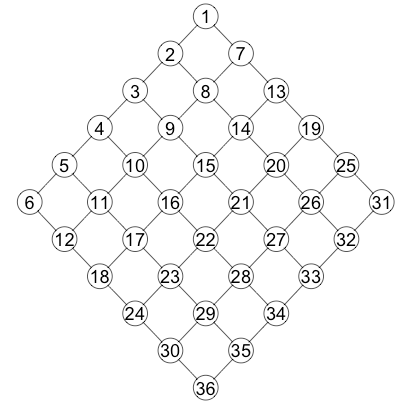### Graph visualization in R

It sometimes happens, that solving a simple connected task requires much more time, than just solving the core problem itself. I happened to me when I decided to visualize graphs in R.

The setting of the problem is the following:
• weighted graphs specified by an adjacency matrix,
• about 50 vertices, many different graphs on the same set of vertices.

Ok, first of all, if you really want to see the results fast and you can read them from adjacency matrix A, just visualize it with:
image(A)
Easy? Almost yes, but because image coordinates are different from the matrix coordinates, you will need to play around with dimensions:
image(A[nrow(A):1,])
Already better. But I faced a situation where discretization of the colors showed me only large entries of A. So if you want to be sure you see everything define the number of colors:
ncolors = length(unique(c(A))) + 1
image(A
[nrow(A):1,], axes=0, col=terrain.colors(ncolors))
Good, that shows something reasonable.Example adjacency matrix

#### Rgraphviz

The most advanced and popular library for graph visualization is the one called "Rgraphviz". The first step is installation.
source("http://bioconductor.org/biocLite.R")
biocLite("Rgraphviz")
library("Rgraphviz")
Yeah, it is not from CRAN, it is a third-party implementation by Bioconductor. If you get errors like "tar: Failed to set default locale" in Mac OS X, try typing the following line in the terminal (the system one, not the R one):
defaults write org.R-project.R force.LANG en_US.UTF-8
And restart R.

Good, you graph is then created with:
gR = graphNEL(nodes=V)
And all the edges are added with the cycle over the adjacency matrix:
if (A[i,j] > 0) { gR = addEdge(V[i], V[j], gR) }
To magically create a layout and plot the graph use:
gR = layoutGraph(gR)
renderGraph(gR)Visualized graph

And here comes the first problem. It took me quite some time to color the edges from white to black depending on the weight of the edge. The only solution I found is the following:
color = paste('gray', round(100*(1-A[i,j])), sep='')
edge_name = paste(V[i], '~', V[j], sep='')
edgeRenderInfo(gR)\$col[edge_name] = colorVisualized graph with weights
Looks strange, but works. In a similar way other properties of the edges can be changed (the name of the edge between nodes "A" and "B" is "A~B").

Good, now I want to plot many graphs with the same set of nodes, but different edges. I need that in order to easily perform the comparison of the results. And this fails completely: the layout is so clever, that it changes all the time even if we remove only a couple of edges.Layout is changing as we change the graph
And this is the problem I did not overcome. The only solution I managed to come up with is to memorize all the node coordinates of already visualized graph and to set the minimum and the maximum coordinates for every node when rendering all the others.

#### igraph

Less advanced, yet simple and beautiful package "igraph" appeared to perfectly suit all my needs. To be short, here is the code that creates a graph from adjacency matrix and memorizes the layout:
gR = graph.adjacency(A, mode="upper", weighted=TRUE, diag=FALSE)
l = layout.fruchterman.reingold(gR)
There are also different possible algorithms to generate layouts, such as: random, circle, sphere, kamada.kawai, reingold.tilford and others.

Now in order to plot a graph we just need to write:
plot.igraph(gR, vertex.label=NA, vertex.size=10, layout=l, edge.color="black", edge.width=1.5*E(net)\$weight)Graph visualization with igraph
That is it. Here not the color controls the appearance of the edge, but the width of the edge. And now as we have the layout stored in l, we can access it for every edge set.Different edge set, same layout

1.2.3.